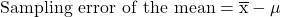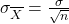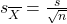Sampling and Estimation | IFT World
IFT Notes for Level I CFA® Program
IFT Notes for Level I CFA® Program

# Part 1

## 1. Introduction

A sample is a subset of a population. We can study a sample to infer conclusions about the population itself. For example, if all the stocks trading in the US are considered a population, then indices such as the S&P 500 are samples. We can look at the performance of the S&P 500 and draw conclusions about how all stocks in the US are performing. This process is known as sampling and estimation.

## 2. Sampling Methods

There are various methods for obtaining information on a population through samples. The information we obtain usually concerns a parameter, a quantity used to describe a population. To estimate a parameter, we use sample statistics. A statistic is a quantity used to describe a sample.

There are two reasons why sampling is used:

• Time saving: In many cases it will be very time consuming to examine every member of the population.
• Monetary saving: In some cases, examining every member of the population becomes economically inefficient.

There are two types of sampling methods:

• Probability sampling: Every member of the population has an equal chance of being selected. Therefore, the sample created is representative of the population.
• Non-probability sampling: Every member of the population may not have an equal chance of being selected. This is because sampling depends on factors such as the sampler’s judgement or the convenience to access data. Therefore, the sample created may not be representative of the population.

All else equal, the probability sampling method is more accurate and reliable as compared to the non-probability sampling method.

In the subsequent sections, we will discuss the following sampling methods:

• Probability sampling
• Simple random sampling
• Systematic sampling
• Stratified random sampling
• Cluster sampling
• Non-probability sampling
• Convenience sampling
• Judgement sampling

### 2.1 Simple Random Sampling

Simple random sampling is the process of selecting a sample from a larger population in such a way that each member of the population has the same probability of being included in the sample.

Sampling distribution

If we draw samples of the same size several times and calculate the sample statistic, the sample statistic will be different each time. The distribution of values of the sample statistic is called a sampling distribution.

For example, say you select 100 stocks from a universe of 10,000 stocks and calculate the average annual returns of these 100 stocks. Let’s say you get an average return of 15%. You repeat this process with a second sample of 100 stocks. This time, you get an average return of 14%. You keep repeating this process and each time you get a different average return. The distribution of these sample average returns is called a sampling distribution.

Sampling error

Sampling error is the difference between a sample statistic and the corresponding population parameter. The sampling error of the mean is given by:For example, let’s say you want to estimate the average returns of 10,000 stocks. You draw a sample of 100 stocks and calculate the average return of these 100 stocks as 15%. However, the actual average of the 10,000 stocks was 12%. Then the sampling error = 15% – 12% = 3%.

Systematic sampling: In this technique, we select every kth member of the population until we have a sample of the desired size. Samples created using this technique should be approximately random.

Instructor’s Note: Researchers calculate the sampling interval ‘k’ by dividing the entire population size by the desired sample size.

### 2.2 Stratified Random Sampling

In stratified random sampling, the population is divided into subgroups based on one or more distinguishing characteristics. Samples are then drawn from each subgroup, with sample size proportional to the size of the subgroup relative to the population. Finally, samples from each subgroup are pooled together to form a stratified random sample.

The advantage of stratified random sampling is that the sample will have the same distribution of key characteristics as the overall population. This can help reduce the sampling error. Stratified random sampling therefore produces more precise parameter estimates than simple random sampling

For example, you divide the universe of 10,000 stocks as per their market capitalization such that you have 5,000 large cap stocks, 3,000 mid cap stocks, and 2,000 small cap stocks. In stratified random sampling, to select a total sample of 100 stocks, you will randomly select 50 large cap stocks, 30 mid cap stocks, and 20 small cap stocks and pool all these samples together to form a stratified random sample.

Example

Paul wants to categorize publicly listed stocks for his research project. He first divides the stocks into 15 industries. Then from each industry, he categorizes companies into three groups: small, medium, large. Finally, he divides these into value versus growth stocks. How many cells or strata does the sampling plan entail?

1. A. 20
2. B. 45
3. C. 90

Solution:

C is correct. This is an application of the multiplication rule of counting. The total number of cells is the product of 15, 3, and 2. Thus the answer is 90.

### 2.3 Cluster Sampling

Cluster sampling is similar to stratified random sampling as it also requires the population to be divided into subpopulation groups, called clusters. Each cluster is essentially a mini-representation of the entire population. Then some random clusters are chosen as a whole for sampling.

Instructor’s Note: Clusters are generally based on natural groups separating the population. For example, you might be able to divide your data into natural groupings like city blocks, voting districts, or school districts.

The main difference between cluster sampling and stratified random sampling is that in cluster sampling, the whole cluster is selected; and not all clusters are included in the sample. In stratified random sampling, however, only a few members from each stratum are selected; but all strata are included in the sample.

The difference between simple random sampling, stratified random sampling, and cluster sampling is illustrated in the figure below:As compared to SRS and stratified sampling, cluster sampling is less accurate because the chosen sample may be less representative of the entire population. However, this method is the most time-efficient and cost-efficient amongst the three.

### 2.4 Non-Probability Sampling

The two major types of non-probability sampling methods are:

• Convenience sampling: In this method, the researcher selects members from a population based on how easy it is to access the member i.e., data is collected from a conveniently available pool of respondents. The disadvantage of this method is that the sample selected may not be representative of the entire population. The advantage is that data can be collected quickly and at a low cost. Hence this method is particularly suitable for small-scale pilot studies.
• Judgmental sampling: In this method, the researcher uses his knowledge and professional judgment to selectively handpick members from the population. The disadvantage of this method is that the sampling may be impacted by the researcher’s bias and the results may be skewed. The advantage of this method is that it allows the researcher to directly go to the target population of interest. For example, when auditing financial statements, experienced auditors can use their professional judgment to select important accounts or transactions that can provide sufficient audit coverage.

### 2.5 Sampling from Different Distributions

Instructor’s Note: This section does not contain testable concepts. The core point is presented below.

In addition to selecting an appropriate sampling method, researchers also need to be careful when sampling from a population that is not under one single distribution. In such cases, the larger population should be divided into smaller parts, and samples should be drawn from the smaller parts separately.

## 3. The Central Limit Theorem and Distribution of the Sample Mean

The sample mean is a random variable with a probability distribution known as the statistic’s sampling distribution. To understand this concept, consider the following population: last year’s returns on every stock traded in the United States. We are interested in the mean return of all stocks but do not have time to calculate the population mean.  Hence, we draw a sample of 50 stocks and compute the sample mean. We then draw another sample of 50 stocks and compute the sample mean. This exercise can be repeated several times giving us a distribution of sample means. This distribution is called the statistic’s sampling distribution. The central limit theorem, explained below, helps us understand the sampling distribution of the mean.

### 3.1 The Central Limit Theorem

According to the central limit theorem, if we draw a sample from a population with a mean µ and a variance σ2, then the sampling distribution of the sample mean:

• will be normally distributed (irrespective of the type of distribution of the original population).
• will have a mean of µ.
• will have a variance of σ2/n.

For example, suppose the average return of the universe of 10,000 stocks is 12% and its standard deviation is 10%. Through central limit theorem we can conclude that if we keep drawing samples of 100 stocks and plot their average returns, we will get a sampling distribution that will be normally distributed with mean = 12% and variance of 102/100 = 1%.

### 3.2 Standard Error of the Sample Mean

The standard deviation of the distribution of the sample means is known as the standard error of the sample mean.

When we know the population standard deviation, the standard error of the sample mean can be calculated as:When we do not know the population standard deviation (σ) we can use the sample standard deviation (s) to estimate the standard error of the sample mean:Example

The mean of a population is 12 and the standard deviation is 3. Given that the population comprises of 64 observations, what is the standard error of the sample mean?

1. A. 0.375
2. B. 0.378
3. C. 0.667

Solution:

A is correct.  Standard Error = σ/√n = 3/√64 = 0.375